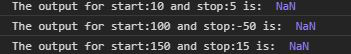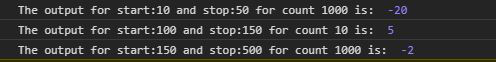# d3.tickIncrement() Function

• Last Updated : 29 May, 2021

The tickIncrement() function of D3.js is used whenever one needs to be sure that the start is always less than the stop where the start and stop are given as a parameter in this function. If the tick step for the given start, stop and count is less than one then it returns the negative inverse tick step.

Syntax:

`d3.tickIncrement(start, stop, count)`

Parameter: This function accepts three parameters as mentioned above and describe below.

• start: It is the starting value from where we want the array element, it is inclusive.
• stop: It is the starting value to which we want the array element to be, it is inclusive.
• count: It is the number of elements we want in a given start and stop range.

Return value: It returns an integer.

Example 1: When start is greater than stop:

## HTML

 `` `<``html` `lang``=``"en"``>`   `<``head``>` `    ``<``meta` `charset``=``"UTF-8"``>` `    ``<``meta` `name``=``"viewport"` `content``=` `        ``"width=device-width, initial-scale=1.0"``>` `    ``<``title``>Document` ``   `<``body``>` `    ``` `    ``<``script` `type``=``"text/javascript"` `        ``src``=``"https://d3js.org/d3.v4.min.js"``>` `    ``` `    `  `    ``<``script``>`   `        ``// What ever the count is it will ` `        ``// always give NaN as output because` `        ``// stop is less than start` `        ``let s = d3.tickIncrement(10, 5, 1);` `        ``console.log("The output for start:10"` `                    ``+ " and stop:5 is: ", s);`   `        ``s = d3.tickIncrement(100, -50, 1);` `        ``console.log("The output for start:100"` `                    ``+ " and stop:-50 is: ", s);`   `        ``s = d3.tickIncrement(100, 5, 1);` `        ``console.log("The output for start:150"` `                    ``+ " and stop:15 is: ", s);` `    ``` ``   ``

Output:Example 2: When the tick step is less than one:

## HTML

 `` `<``html` `lang``=``"en"``>`   `<``head``>` `    ``<``meta` `charset``=``"UTF-8"``>` `    ``<``meta` `name``=``"viewport"` `content``=` `        ``"width=device-width, initial-scale=1.0"``>` ``   `<``body``>` `    ``` `    ``<``script` `type``=``"text/javascript"` `        ``src``=``"https://d3js.org/d3.v4.min.js"``>` `    ``` `    `  `    ``<``script``>`   `        ``// If count is so large such that ` `        ``// the tick value is less than one` `        ``// it gives the negative number` `        ``let s = d3.tickIncrement(10, 50, 1000);` `        ``console.log("The output for start:10 and"` `            ``+ " stop:50 for count 1000 is: ", s);`   `        ``s = d3.tickIncrement(100, 150, 10);` `        ``console.log("The output for start:100 and"` `            ``+ " stop:150 for count 10 is: ", s);`   `        ``s = d3.tickIncrement(100, 500, 1000);` `        ``console.log("The output for start:150 and"` `            ``+ " stop:500 for count 1000 is: ", s);` `    ``` ``   ``

Output:My Personal Notes arrow_drop_up
Recommended Articles
Page :